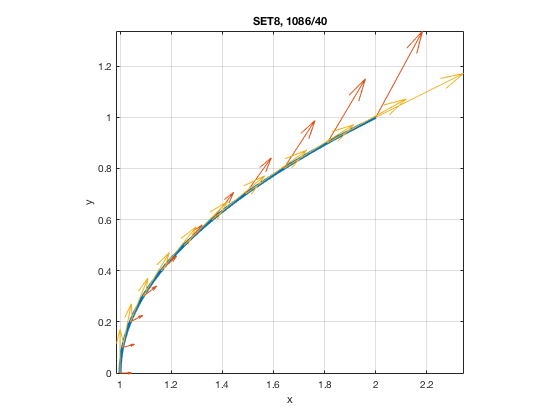# MATH 251: Calculus 3, SET8

## 16: Vector Calculus

### 16.2: Line Integrals

These problems are done with the CAS. See Hand Solutions for details.

#### 1. [1084/8]

syms F r1 r2 t x y real
% Vector field, quarter circular arc, line segment:
F = [x^2 y^2], r1 = [2*cos(t) 2*sin(t)], r2 = [0 2] + t*([4 3]-[0 2])
F =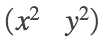r1 =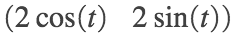r2 =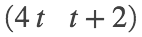cFr1 = subs(F, [x y], r1) % Compose F with r1.
cFr1 =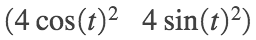Dr1 = diff(r1,t) % Differentiate r1 w.r.t. t.
Dr1 =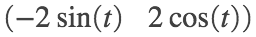cFr2 = subs(F, [x y], r2) % Compose F with r2.
cFr2 =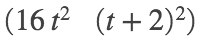Dr2 = diff(r2,t) % Differentiate r2 w.r.t. t.
Dr2 =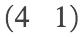intg1 = dot(cFr1, Dr1) % 1st integrand
intg1 =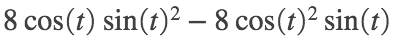intg2 = dot(cFr2, Dr2) % 2nd integrand
intg2 =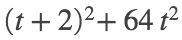I1 = int(intg1, t, 0, pi/2) % line integral of vector field along quarter-circular arc
I1 = 0
I2 = int(intg2, t, 0, 1) % line integral of vector field along line segment
I2 =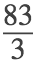I = I1 + I2 % Add up portions.
I =I_appx = double(I) % Approximate.
I_appx = 27.6667
%
% Path
figure
fplot(2*cos(t), 2*sin(t), [0 pi/2], 'LineWidth', 3); grid on; hold on
fplot(4*t, t+2, [0 1], 'LineWidth', 3)
axis equal; xticks(0:4); yticks(0:3)
xlabel('x'); ylabel('y')
title('SET8, 1084/8')
% Vector field
[x y] = meshgrid(0:0.5:4, 0:0.5:3);
u = x.^2; v = y.^2;
quiver(x,y,u,v)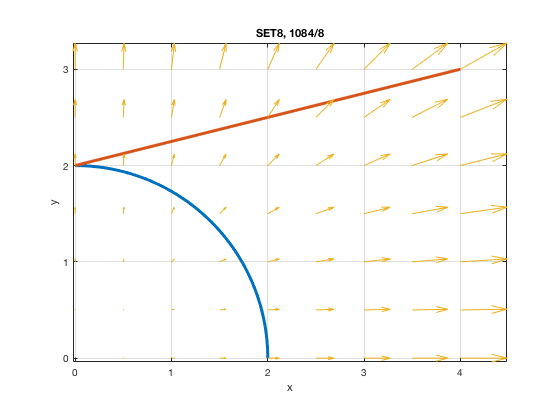#### 2. [1084/12]

syms f r t x y z real
f = x^2 + y^2 + z^2, r = [t cos(2*t) sin(2*t)] % scalar field, helix (a space curve)
f =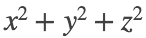r =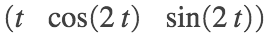cfr = simplify( subs(f, [x y z], r) ) % Compose f with r.
cfr =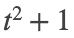Dr = diff(r,t) % Differentiate r w.r.t. t.
Dr =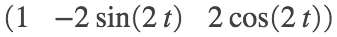mDr = simplify( sqrt(sum(Dr.^2)) ) % magnitude of r'
mDr =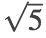intg = cfr * mDr % integrand
intg =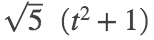I = int(intg, t, 0, 2*pi) % line integral of scalar field along space curve
I =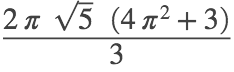I_appx = double(I) % Approximate.
I_appx = 198.9353
% Path
figure
fplot3(r(1), r(2), r(3), [0 2*pi], 'LineWidth', 2); grid on
axis equal; xticks(0:6); yticks(-1:1); zticks(-1:1)
xlabel('x'); ylabel('y'); zlabel('z')
title('SET8, 1084/12')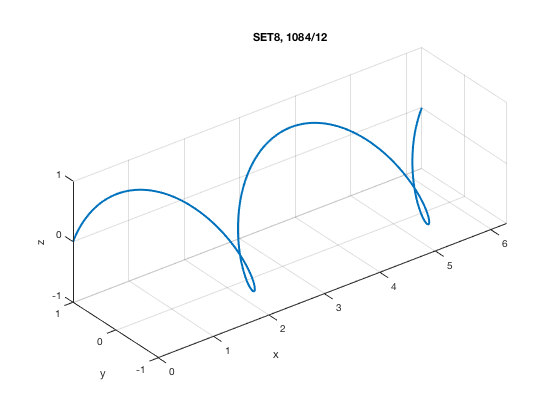#### 3. [1085/20]

syms F r t x y z real
F = [x+y^2 x*z y+z], r = [t^2 t^3 -2*t] % vector field, space curve
F =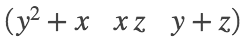r =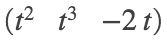cFr = subs(F, [x y z], r) % Compose F with r.
cFr =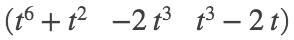Dr = diff(r,t) % Differentiate r w.r.t. t.
Dr =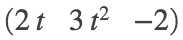intg = expand( dot(cFr, Dr) ) % integrand
intg =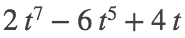I = int(intg, t, 0, 2) % line integral of vector field along space curve
I = 8
%
% Path
figure
fplot3(r(1), r(2), r(3), [0 2], 'LineWidth', 3); grid on; hold on
axis equal; xticks(0:2:4); yticks(0:2:8); zticks(-4:2:0)
xlabel('x'); ylabel('y'); zlabel('z')
title('SET8, 1085/20'); view(-31,5)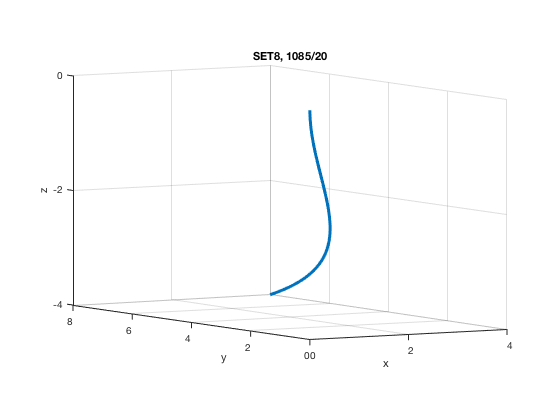#### 4. [1085/22]

syms F r t x y z real
F = [x y x*y], r = [cos(t) sin(t) t] % vector field, space curve
F =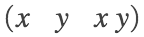r =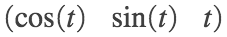cFr = subs(F, [x y z], r) % Compose F with r.
cFr =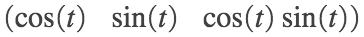Dr = diff(r,t) % Differentiate r w.r.t. t.
Dr =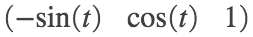intg = expand( dot(cFr, Dr) ) % integrand
intg =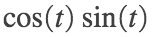I = int(intg, t, 0, pi) % line integral of vector field along space curve
I = 0
%
% Path
figure
fplot3(r(1), r(2), r(3), [0 pi], 'LineWidth', 3); grid on; hold on
axis equal; xticks(-1:1); yticks(0:1); zticks(0:3)
xlabel('x'); ylabel('y'); zlabel('z')
title('SET8, 1085/22'); view(60,60)
% Vector field arrows along the path
t = linspace(0,pi,11); x = cos(t); y = sin(t); z = t;
u = x; v = y; w = x.*y;
quiver3(x,y,z,u,v,w)
% Tangent vectors along path
U = -sin(t); V = cos(t); W = 1+0*t;
quiver3(x,y,z,U,V,W)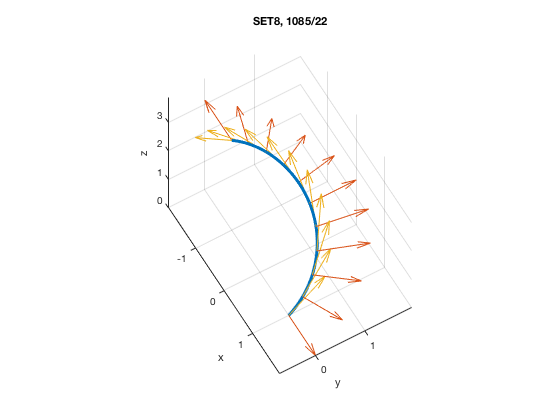#### 5. [1085/26]

syms f r t x y z real
f = z*log(x+y), r = [1+3*t 2+t^2 t^4] % scalar field, space curve
f =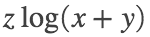r =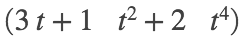cfr = simplify( subs(f, [x y z], r) ) % Compose f with r.
cfr =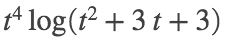Dr = diff(r,t) % Differentiate r w.r.t. t.
Dr =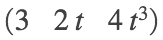mDr = simplify( sqrt(sum(Dr.^2)) ) % magnitude of r'
mDr =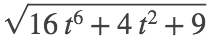intg = cfr * mDr % integrand
intg =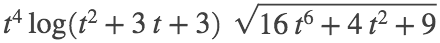I = int(intg, t, -1, 1) % line integral of scalar field along space curve
I =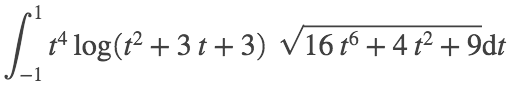I_appx = double(I) % Approximate.
I_appx = 1.7260
% Path
figure
fplot3(r(1), r(2), r(3), [-1 1], 'LineWidth', 2); grid on
axis equal; xticks(-2:4); yticks(2:3); zticks(0:1)
xlabel('x'); ylabel('y'); zlabel('z')
title('SET8, 1085/26')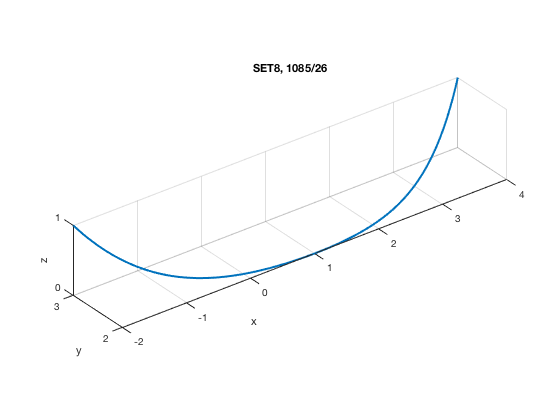#### 6. [1085/28]

syms F r t x y real
F = [x y] / sqrt(x^2 + y^2), r = [t 1+t^2] % vector field, plane curve
F =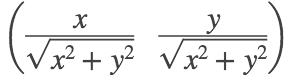r =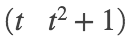cFr = subs(F, [x y], r) % Compose F with r.
cFr =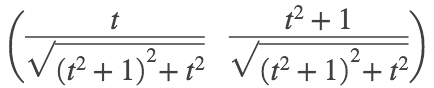Dr = diff(r,t) % Differentiate r w.r.t. t.
Dr =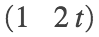intg = expand( dot(cFr, Dr) ) % integrand
intg =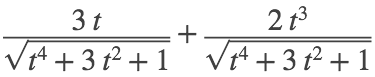I = int(intg, t, -1, 1) % line integral of vector field along plane curve
I = 0
I_appx = double(I) % Approximate.
I_appx = 0
%
% Path
figure
fplot(r(1), r(2), [-1 1], 'LineWidth', 5); grid on; hold on
axis equal
xlabel('x'); ylabel('y')
title('SET8, 1085/28')
% Vector field arrows along the path
t = linspace(-1,1,11); x = t; y = 1+t.^2;
u = x./sqrt(x.^2 + y.^2); v = y./sqrt(x.^2 + y.^2);
quiver(x,y,u,v)
% Tangent vectors along path
U = 1+0*t; V = 2*t;
quiver(x,y,U,V)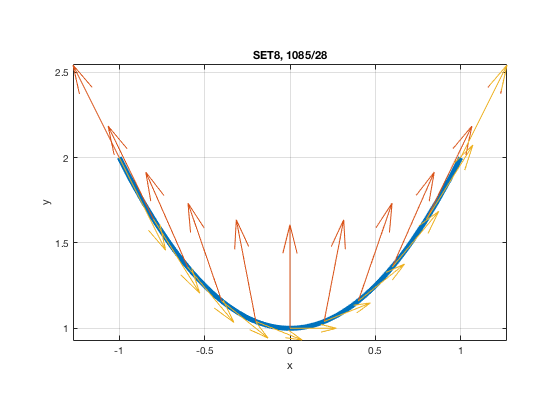#### 7. [1085/30]

syms F r t x y z real
F = [x -z y], r = [2*t 3*t -t^2] % vector field, space curve
F =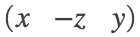r =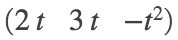cFr = subs(F, [x y z], r) % Compose F with r.
cFr =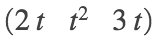Dr = diff(r,t) % Differentiate r w.r.t. t.
Dr =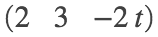intg = expand( dot(cFr, Dr) ) % integrand
intg =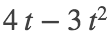I = int(intg, t, -1, 1) % line integral of vector field along space curve
I =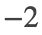%
% Path
figure
fplot3(r(1), r(2), r(3), [-1 1], 'LineWidth', 3); grid on; hold on
axis equal; xticks(-2:2); yticks(-3:3); zticks(-2:0)
xlabel('x'); ylabel('y'); zlabel('z')
title('SET8, 1085/30'); view(58,16)
% Vector field arrows along the path
t = linspace(-1,1,11); x = 2*t; y = 3*t; z = -t.^2;
u = x; v = -z; w = y;
quiver3(x,y,z,u,v,w)
% Tangent vectors along path
U = 2+0*t; V = 3+0*t; W = -2*t;
quiver3(x,y,z,U,V,W)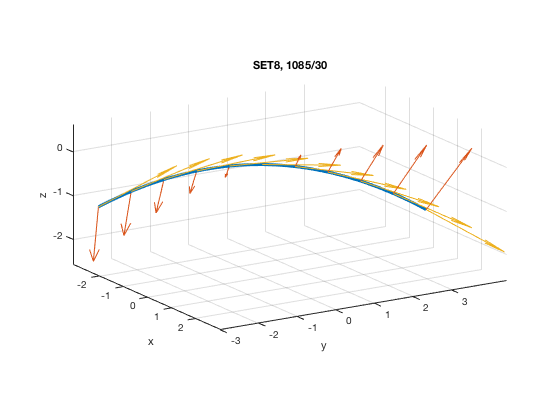#### 8. [1085/34]

syms a d k r t x y positive % d = delta = density
d = k*x*y, r = [a*cos(t) a*sin(t)] % scalar field, plane curve
d =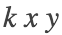r =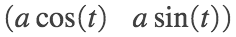cdr = simplify( subs(d, [x y], r) ) % Compose d with r.
cdr =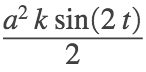Dr = diff(r,t) % Differentiate r w.r.t. t.
Dr =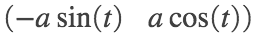mDr = simplify( sqrt(sum(Dr.^2)) ) % magnitude of r'
mDr = a
intg = cdr * mDr % integrand
intg =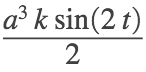m = int(intg, t, 0, pi/2) % mass
m =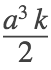CM = 1/m * int(intg*r, t, 0, pi/2) % CM: center of mass; here r = [x(t) y(t)]
CM =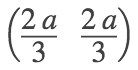% curve
figure
fplot(cos(t), sin(t), 'LineWidth', 2); grid on; hold on
axis equal; axis([0 1.5 0 1.5]); xticks(0:1); yticks(0:1)
xlabel('x'); ylabel('y')
xticks([0 1]); xticklabels({'0','a'})
yticks([0 1]); yticklabels({'0','a'})
title('SET8, 1085/34')
plot(2/3, 2/3, 'm+', 'MarkerSize', 14)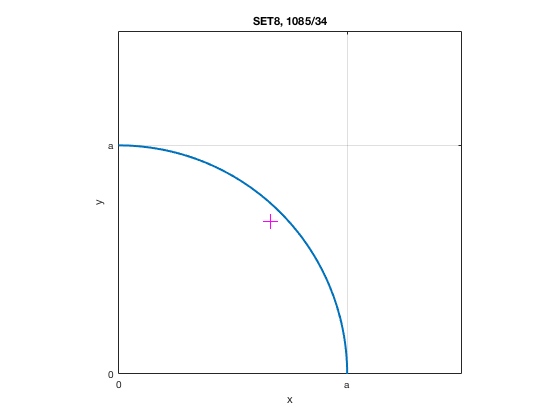#### 9. [1086/36]

syms d r t x y z % d = delta = density
d = x^2 + y^2 + z^2, r = [t cos(t) sin(t)] % scalar field, space curve
d =r =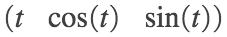cdr = simplify( subs(d, [x y z], r) ) % Compose d with r.
cdr =Dr = diff(r,t) % Differentiate r w.r.t. t.
Dr =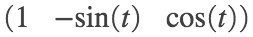mDr = simplify( sqrt(sum(Dr.^2)) ) % magnitude of r'
mDr =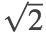intg = cdr * mDr % integrand
intg =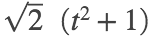m = int(intg, t, 0, 2*pi) % mass
m =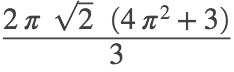m_appx = double(m) % g
m_appx = 125.8178
CM = 1/m * int(intg*r, t, 0, 2*pi) % CM: center of mass; here r = [x(t) y(t) z(t)]
CM =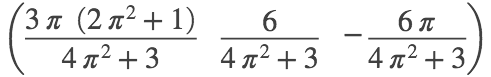CM_appx = double(CM) % components in cm
CM_appx = 1×3
4.6015 0.1412 -0.4437
% curve
figure
fplot3(t, cos(t), sin(t), [0 2*pi], 'LineWidth', 2); grid on; hold on
axis equal; % axis([0 1.5 0 1.5]); xticks(0:1); yticks(0:1)
xlabel('x'); ylabel('y'); zlabel('z')
title('SET8, 1085/36'); view(23,28)
plot3(4.6015, 0.1412, -0.4437, 'm+', 'MarkerSize', 14)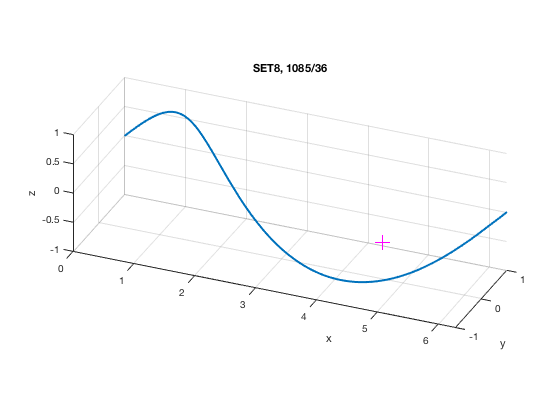#### 10. [1086/40]

syms F r t x y real
F = [x^2 y*exp(x)], r = [1+t^2 t] % vector field, plane curve
F =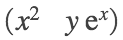r =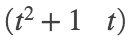cFr = subs(F, [x y], r) % Compose F with r.
cFr =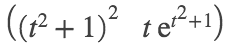Dr = diff(r,t) % Differentiate r w.r.t. t.
Dr =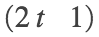intg = expand( dot(cFr, Dr) ) % integrand
intg =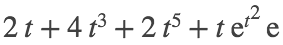work = expand( int(intg, t, 0, 1) ) % work done by force field on a particle along a plane curve
work =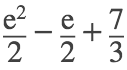work_appx = double(work) % Approximate.
work_appx = 4.6687
%
% Path
figure
fplot(r(1), r(2), [0 1], 'LineWidth', 3); grid on; hold on
axis equal; % axis([-1.2 1.2 1 2.2])
xlabel('x'); ylabel('y')
title('SET8, 1086/40')
% Vector field arrows along the path
t = linspace(0,1,11); x = 1 + t.^2; y = t;
u = x.^2; v = y.*exp(x);
quiver(x,y,u,v)
% Tangent vectors along path
U = 2*t; V = 1+0*t;;
quiver(x,y,U,V)